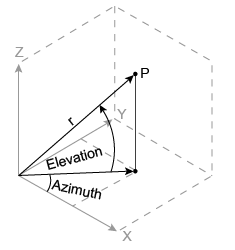# sph2cart

Transform spherical coordinates to Cartesian

## Syntax

``[x,y,z] = sph2cart(azimuth,elevation,r)``

## Description

example

````[x,y,z] = sph2cart(azimuth,elevation,r)` transforms corresponding elements of the spherical coordinate arrays `azimuth`, `elevation`, and `r` to Cartesian, or xyz, coordinates.```

## Examples

collapse all

Convert the spherical coordinates defined by corresponding entries in the matrices `az`, `el`, and `r` to Cartesian coordinates `x`, `y`, and `z`. These points correspond to the eight vertices of a cube.

`az = [0.7854 0.7854 -0.7854 -0.7854; 2.3562 2.3562 -2.3562 -2.3562]`
```az = 2×4 0.7854 0.7854 -0.7854 -0.7854 2.3562 2.3562 -2.3562 -2.3562 ```
`el = [0.6155 -0.6155 0.6155 -0.6155; 0.6155 -0.6155 0.6155 -0.6155]`
```el = 2×4 0.6155 -0.6155 0.6155 -0.6155 0.6155 -0.6155 0.6155 -0.6155 ```
`r = 1.7321*ones(2,4)`
```r = 2×4 1.7321 1.7321 1.7321 1.7321 1.7321 1.7321 1.7321 1.7321 ```
`[x,y,z] = sph2cart(az,el,r)`
```x = 2×4 1.0000 1.0000 1.0000 1.0000 -1.0000 -1.0000 -1.0000 -1.0000 ```
```y = 2×4 1.0000 1.0000 -1.0000 -1.0000 1.0000 1.0000 -1.0000 -1.0000 ```
```z = 2×4 1.0001 -1.0001 1.0001 -1.0001 1.0001 -1.0001 1.0001 -1.0001 ```

## Input Arguments

collapse all

Azimuth angle, specified as a scalar, vector, matrix, or multidimensional array. `azimuth`, `elevation`, and `r` must be the same size, or have sizes that are compatible (for example, `azimuth` is an `M`-by-`N` matrix, `elevation` is a scalar, and `r` is a scalar or `1`-by-`N` row vector). For more information, see Compatible Array Sizes for Basic Operations.

`azimuth` is the counterclockwise angle in the x-y plane measured in radians from the positive x-axis.

Data Types: `single` | `double`
Complex Number Support: Yes

Elevation angle, specified as a scalar, vector, matrix, or multidimensional array. `azimuth`, `elevation`, and `r` must be the same size, or have sizes that are compatible.

`elevation` is the elevation angle in radians from the x-y plane.

Data Types: `single` | `double`
Complex Number Support: Yes

Radius, specified as a scalar, vector, matrix, or multidimensional array. `azimuth`, `elevation`, and `r` must be the same size, or have sizes that are compatible.

The length units of `r` can be arbitrary, and the output arrays `x`, `y`, and `z` use the same units.

Data Types: `single` | `double`
Complex Number Support: Yes

## Output Arguments

collapse all

Cartesian coordinates, returned as arrays.

## Algorithms

The mapping from spherical coordinates to three-dimensional Cartesian coordinates is

```x = r .* cos(elevation) .* cos(azimuth) y = r .* cos(elevation) .* sin(azimuth) z = r .* sin(elevation)```## Version History

Introduced before R2006a